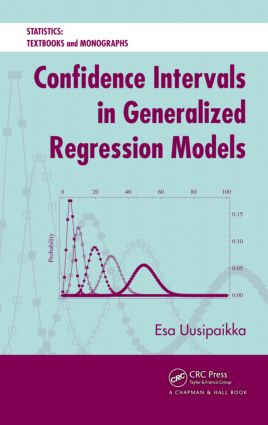# Confidence Intervals in Generalized Regression Models

## 1st Edition

Chapman and Hall/CRC

328 pages | 91 B/W Illus.

Hardback: 9781420060270
pub: 2008-07-25
SAVE ~\$27.00
Currently out of stock
\$135.00
\$108.00
x
eBook (VitalSource) : 9780429148545
pub: 2008-07-25
from \$67.50

FREE Standard Shipping!

### Description

A Cohesive Approach to Regression Models

Confidence Intervals in Generalized Regression Models introduces a unified representation—the generalized regression model (GRM)—of various types of regression models. It also uses a likelihood-based approach for performing statistical inference from statistical evidence consisting of data and its statistical model.

Provides a Large Collection of Models

The book encompasses a number of different regression models, from very simple to more complex ones. It covers the general linear model (GLM), nonlinear regression model, generalized linear model (GLIM), logistic regression model, Poisson regression model, multinomial regression model, and Cox regression model. The author also explains methods of constructing confidence regions, profile likelihood-based confidence intervals, and likelihood ratio tests.

Uses Statistical Inference Package to Make Inferences on Real-Valued Parameter Functions

Offering software that helps with statistical analyses, this book focuses on producing statistical inferences for data modeled by GRMs. It contains numerical and graphical results while providing the code online.

Introduction

Likelihood-Based Statistical Inference

Statistical evidence

Statistical inference

Likelihood concepts and law of likelihood

Likelihood-based methods

Profile likelihood-based confidence intervals

Likelihood ratio tests (LRTs)

Maximum likelihood estimate (MLE)

Model selection

Generalized Regression Model

Examples of regression data

Definition of generalized regression models (GRMs)

Special cases of GRM

Likelihood inference

MLE with iterative reweighted least squares

Model checking

General Linear Model

Definition of the general linear model (GLM)

Estimate of regression coefficients

Test of linear hypotheses

Confidence regions and intervals

Model checking

Nonlinear Regression Model

Definition of the nonlinear regression model

Estimate of regression parameters

Approximate distribution of LRT statistic

Profile likelihood-based confidence region

Profile likelihood-based confidence interval

LRT for a hypothesis on finite set of functions

Model checking

Generalized Linear Model

Definition of generalized linear model (GLIM)

MLE of regression coefficients

Binomial and Logistic Regression Models

Data

Binomial distribution

Likelihood inference

Logistic regression model

Nonlinear binomial regression model

Poisson Regression Model

Data

Poisson distribution

Likelihood inference

Log-linear model

Multinomial Regression

Data

Multinomial distribution

Likelihood function

Logistic multinomial regression model

Proportional odds regression model

Other Generalized Linear Regressions Models

Negative binomial regression model

Gamma regression model

Other Generalized Regression Models

Weighted GLM

Weighted nonlinear regression model

Quality design or Taguchi model

Cox regression model

Appendix A: Data Sets

Appendix B: Notation Used for Statistical Models

Bibliographic notes appear at the end of each chapter.